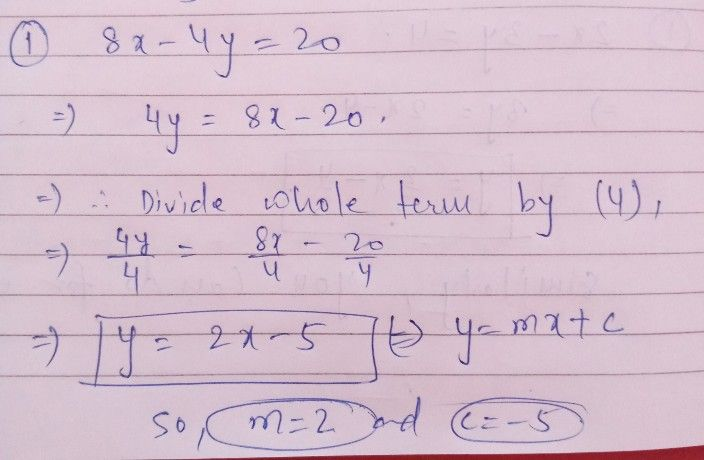Symbol
ProblemRewrite each $01$ the following equations in $slope-intercep$ $formy=mx+b,and$ identify $Nr$ $y-intθrCept$ Show each step. the slope $andy-1n$ $1.8x-4y=20$ $2.2x+3y=12$ $3,2x+y=-11$ $4.3y=4x-27$ $5.x-4y=8$
7th-9th grade
Other
Search count: 108
SolutionQanda teacher - aftabalamsimilarly you can do for everyone
please provide some gift coins and also please evaluate and like ?